### Get Best and Most Reliable Symmetric and Asymmetric Encryption Algorithms Assignment Help Service at Expertsminds!!

Home   Course   Symmetric and Asymmetric Encryption Algorithms Assignment Help
Previous << || >> Next

Assignment - Write a report about Four types of symmetric and asymmetric Encryption algorithms.

Introduction about symmetric and asymmetric Encryption. Discuss the most famous Algorithms (two for each type). Support your report with examples and applications are using these algorithms. Explain about the security features that you added to your system( in the first part) & include the code.

ORDER NEW SYMMETRIC AND ASYMMETRIC ENCRYPTION ALGORITHMS ASSIGNMENT AT NOMINAL PRICE!

Introduction

In today's world network attacks are becoming very prominent. To protect the data from intruders' attack it is very important to encrypt the data using various encryption algorithms. There are two types of encryption that is symmetric and asymmetric encryption .Encryption is the technique of converting plain text to coded text. In symmetric encryption single key is used by sender and receiver of the message to encrypt and decrypt the message but in asymmetric encryption two keys are used. From two keys one is public key and the other one is private key .The public key is disclosed to everyone but the private key is kept by the receiver. At the time of encryption public key is used and at the time of decryption private key is used.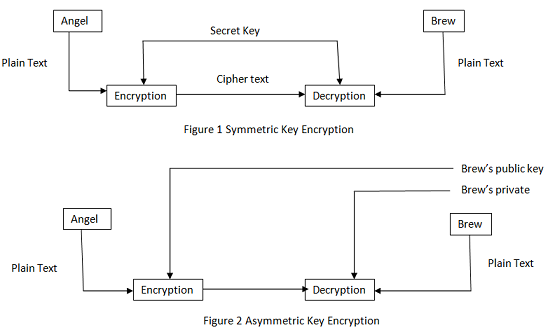In figure 1, Angel sends message to Brew using a secret key which converts the Plain text into cipher text . Using that secret key the receiver of the message Brew coverts the cipher text to plain text. The encryption and decryption are done by the sender and the receiver using the same key. Thus, figure 1 shows symmetric key Encryption.

In figure 2, two keys are used one is public key and the other one is private key .The sender of the message that is Angel encrypts the plain text message using the public key which is disclosed to everyone and the receiver of the message that is Brew decrypts the message using the private key. Since, two keys are used by sender and receiver for the encryption and decryption of the message so, it is asymmetric key encryption.

Algorithms of the Symmetric Encryption

There are a number of symmetric key algorithms available like AES, RC4, DES, RC5 which are used for network security. We will discuss two symmetric key algorithms in this report. One is data encryption standard and the other one is Advanced Encryption standard.

Data Encryption Standard (DES)

This algorithm was invented by IBM and the US government used it the most for non military purposes. The DES algorithm converts 64 bit plain text into 64 bit key. This algorithm divides the plain text into blocks of 32 bits each and uses the same key to encrypt and decrypt these blocks. The 64 bit key is converted to 56 bit key as it discards every 8th bit of the key and uses only 56 bit key.it uses transposition and substitution cipher to encrypt the plain text.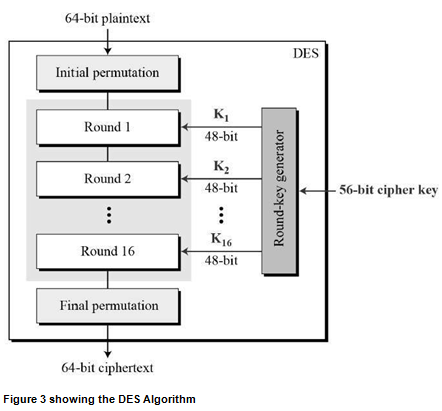The steps involved in Data Encryption standard Algorithm

1) The first step involves performing initial permutation on the plain text. The initial permutation involves only shuffling of the 64 bits. For example the first bit is interchanges with 58th bit and the second bit is interchanged with the 50th bit and so on. The initial permutation is performed on the plain text only once before starting of the rounds.

a) To transform the permutated text into two blocks of 32 bits each, the initial 64 bit key is converted into 56 bit key by ignoring every 8th bit of the permutated block. In this way, the 8 bits are bits are removed from the original permutated text and we get 56 bits text.

b) Now, with the help of compression permutation, we will convert these 56 bits into 48 bits. The 56 bits are divided into two halves of 28 bits each. We will perform circular left rotation on the obtained blocks and select 48 bits from it.

2) After shuffling of the bits using the initial permutation function, the text obtained after permutation is divided into two of 32 bits each. Now, these 32 bits blocks are called left plain text that contains leftmost 32 bits and the right plain text that contains rightmost 32 bits. These two blocks goes through a series of 16 steps of permutation to generate the final key.

a) The right permutation text is expended to 48 bits from 32 bits by dividing 32 bits to 8 blocks containing 4 bits each. Then, these 8 blocks containing 4 bits each are expanded to 6 bit blocks.

b) Then, XOR operation is performed with the right plain text containing 48 bits with 48 bits key obtained after the expansion.

c) After that, S-box substitution is done.

3) Then, 16 bits rounds of encryption are performed with these 32 bits blocks.

4) Then, these two blocks rejoined and final permutation is performed to get the cipher text.

5) We get the cipher text.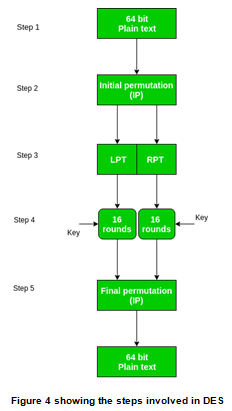Limitations of using Data encryption standard algorithm

1) The DES algorithm works at a very slow speed to generate the encryption keys.

2) It takes a lot of time and money for its operation.

3) DES produces keys of small size.

4) It is vulnerable to attacks of key search.

To overcome the limitations of the DES algorithm a variant of DES algorithm, triple DES was purposed which involves the process of encrypt-decrypt-encrypt.

GET BENEFITTED WITH QUALITY SYMMETRIC AND ASYMMETRIC ENCRYPTION ALGORITHMS ASSIGNMENT HELP SERVICE OF EXPERTSMINDS.COM

Triple Data Encryption Standard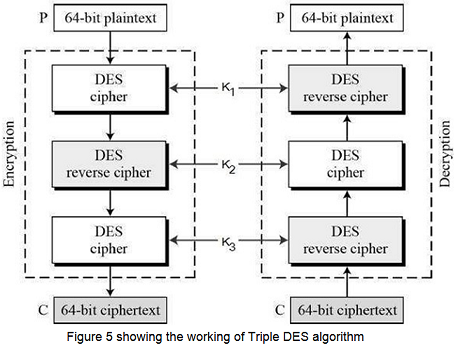The steps involved in the Triple Data Encryption Standard Algorithm

1) As shown in the figure, first of all the plain text is converted into the cipher text using the key k1 that is generated using DES,

2) Then, the output of first step is taken as the input for the next step and the input is decrypted using the key k2.

3) After, decrypting the output in second step, it is again encrypted using the key k3.

4) We will get the cipher text after the third step.

5) The decryption is performed using the reverse process.

The advanced encryption standard algorithm is a algorithm that uses block cipher keys for the process of encryption and decryption. It is six times faster than the data encryption standard algorithm. It also produces large size keys for encryption and it is also not prone to key search attacks. AES algorithm encrypts 128 bit data using 128, 192 and 256 bits keys.AES is faster than DES because performs all the operation on bytes rather than bits. It involves performing of substitution and permutation operations on the plain text. The DES had fixed number of rounds but in AES number of rounds vary according to length of key.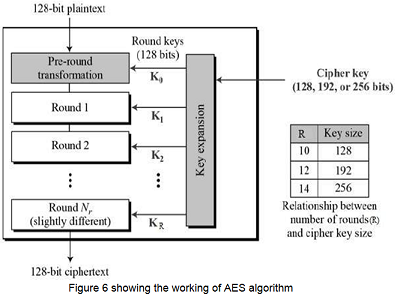Steps involved in the Advanced Encryption Standard (AES)

1) Byte Substitution

The First step of AES algorithm is converting 128 bits of the input into 16 bytes. The conversion is performed using the fixed S-box table. After, conversion we get output in the form of matrix having four columns and four rows.

2) Shift rows

The rows contained in the matrix are shifted to the left and the last row is shifted to the right. We shift rows using following criteria:-

a) The first horizontal line line is kept at its initial position.

b) The second horizontal line is shifted to left by one position.

c) The third horizontal line is shifted to left by two positions.

d) The fourth horizontal line is shifted to left by three positions.

By performing the shift operation on the matrix we get a new matrix containing 16 bytes with four rows and four columns.

3) Mix columns

After shifting the rows, the mathematical operations are performed on each entry of 4 bytes of the matrix which gives a totally new four bytes as a result. So, again we get matrix of 16 bytes with all new bytes.

4) Add round key

The 16 bytes of the obtained matrix are again converted to 128 bits and a XOR operation is performed on these 128 bits using the 128 bits of the round key . if we do not want to perform the further operations then, this step gives us the cipher text otherwise, this is used as input for the further rounds and the 128 bits are again converted to matrix and all the steps are repeated till we do not get final output.

5) The process of decryption requires all the steps in reverse order that is

Mix columns

Shift rows

Byte substitution

Pros of using AES algorithm

1) DES algorithm is much slower than the AES algorithm but AES algorithm provides faster speed.

2) It is not prone to key search attacks.

3) It is more secure than Des algorithm as encryption and decryption are performed separately.

4) It uses different number of rounds for the different key sizes.

5) The operations are performed on the bytes not the bits.

Limitations of AES algorithm

1) It is complex to understand and implement.

2) It requires a large amount of money for its implementation.

ORDER NEW COPY OF SYMMETRIC AND ASYMMETRIC ENCRYPTION ALGORITHMS ASSIGNMENT & GET HIGH QUALITY SOLUTIONS FROM SUBJECT'S TUTORS!

Asymmetric Key Algorithms

There are a number of asymmetric algorithms available. Some of them are RSA ,DSA and Diffie Hellman. We will discuss two algorithms in this report named as RSA and Diffie hellman.

RSA algorithm

This Asymmetric algorithm was invented by Rivest, Shamir and Adleman. It uses two keys one is public key and other one is private for the process of encryption and decryption. The two numbers used for this purpose are e and d.

Steps involved in RSA algorithm

1) First of all two prime numbers are choosen to determine public and private keys. Suppose we choose m and n as numbers. The prime numbers are the ones that are divisible by 1 and itself only.

2) After choosing the prime numbers we find the modulus for encryption and decryption by multiplying the two choosen prime numbers and assign the result to new variable suppose the variable name is g. g=m*n

3) Then, another number s is calculated by multiplying s=(m-1)(n-1).

4) Then, a random integer e and d is choosen so that d*e=1mod s.

5) The numbers g and e are disclosed to public and d and s are kept secret.

6) Now, the sender sends the message by encrypting the message using g and e.

Cipher text =Plaintexte(mod g)

7) The receiver of the message decrypts the message using the private numbers d and s.

Plain text=Ciphertextd(mod g)

Limitation of RSA

1) If the plain text is large number then, it needs to be divided into blocks as the algorithm works only for Plain text less than g.

2) This algorithm is complex to understand.

3) It is very slow for the long messages.

SAVE TOP GRADE USING SYMMETRIC AND ASYMMETRIC ENCRYPTION ALGORITHMS ASSIGNMENT HELP SERVICE OF EXPERTSMINDS.COM!

Example of RSA

Suppose the Jennifer chooses 7 and 11 as the numbers . For these two numbers g is calculated as (7-1)(11-1)=60. Now, Jennifer chooses two keys e and d to be 13 and 37. Now, the ali sends plain text as 5 to Jennifer. She uses the public key13 to encrypt 5.

Plain text=5

C=513=26 mod 77

Cipher text=26

Jennifer receives 26 as the cipher text and uses the key 37 to decrypt the text

Cipher text:26

P=2637=5mod 77

Plain text:5

The pain text 5 sent by Ali is received as Plain text by Jennifer.

Applications

The RSA is used for the purpose of digital signatures and authentication of users to avoid any intruders entry into the system. The RSA algorithm is also used in the cryptography of electronis transfers. It is used to secure data transmission in case of ecommerce.

Diffie Hellman Algorithm

It is a asymmetric key algorithm that uses different keys for encryption and decryption.

Steps involved in Diffie Hellman algorithm

1) The sender and receiver of the message needs to chose two numbers. One number should be large prime number and other one should be any random number. These two numbers need not to be private. These can be disclosed to public.

2) The sender of the message chooses large random number say x and we calculate R1=gx mod p.

3) The receiver of the message chooses another large number say y and we calculate R2= gy mod p.

4) The receiver sends R2 to sender. The value of y is not send.

5) The sender calculates K= (R2)x mod p.

6) The receiver calculates K=(R2)ymod p.

7) From these two values symmetric key for the session K is made.

( gx mod p)ymod p=( gymod p)xmod p=( gx ymod p)

K=( gx ymod p)

Limitation of Diffie Hellman Algorithm

1) The sender and receiver are prone to man in the middle attack.

2) This algorithm is complex to understand.

DO YOU WANT TO EXCEL IN SYMMETRIC AND ASYMMETRIC ENCRYPTION ALGORITHMS ASSIGNMENT - ORDER AT EXPERTSMINDS!

Example

Let George be the sender of the message and Alisha be the receiver of the message. George chooses x=3 and Calculates R1=73mod 23=21

Alisha chooses y=6 and calculates R2=76mod 23=4

George sends the number 21 to Alisha.

Alisha sends the number 4to George.

George calculates the symmetric key=43mod 23=18

Alisha calculates the symmetric key=216mod 23=18

The value of key is same for George and Alisha; gxymod p=718mod 35=18

Application of Diffie Hellman Algorithm

The DHA algorithm is used for the purpose of encrypting messages and digital signatiures . it also encrypts various username and passwords and protect them from intruders attack.

Security features added in the code

1) The code implements the MD5 hashing algorithm to encrypt the message which can protect the disclosure of information in case of intruders attack.The hash key is used to encrypt the message.

2) The code also implements protects the entry of unauthorized users into the system by implementing code of detection of unauthorized login. The users which are there in database of the server only those users can login.

3) All the usernames and passwords of the new users are stored in the database so that if any user forgot or lost his username and password. it can be recovered with the help of database. The stored username can be sent to the email of user using SMTP (Simple mail transfer Protocol).

4) To make the passwords more secure, constraint is imposed on the password that the password length can not be less than 3. It reduces the chances of password guessing attack by the intruder.

5) To authenticate the user the code enforce password integrity . if the user enter wrong password for more than three times then, he will be blocked and he has to send email from registered email id to unblock himself.

DON'T MISS YOUR CHANCE TO EXCEL IN SYMMETRIC AND ASYMMETRIC ENCRYPTION ALGORITHMS ASSIGNMENT! HIRE TUTOR OF EXPERTSMINDS.COM FOR PERFECTLY WRITTEN SYMMETRIC AND ASYMMETRIC ENCRYPTION ALGORITHMS ASSIGNMENT SOLUTIONS!

Code

publicstaticstring stringToMD5Hash(string input)

{

StringBuilder hash = newStringBuilder();

MD5CryptoServiceProvider md5provider = new MD5CryptoServiceProvider();

byte[] bytes = md5provider.ComputeHash(new UTF8Encoding().GetBytes(input));

for (inti = 0; i<bytes.Length; i++)

{

hash.Append(bytes[i].ToString("x2"));

}

returnhash.ToString();

}

protectedvoid Button1_Click(object sender, EventArgs e)

{

if (txtKey.Text== "") {

return; }

intsh = Convert.ToInt32(txtKey.Text);

string value = TextBox1.Text;

char[] c = value.ToCharArray();

for (inti = 0; i<c.Length; i++)

{

var letter = c[i];

letter = (char)(letter + sh);

if (letter >'z')

{

letter = (char)(letter - 26);

}

elseif (letter <'a')

{

letter = (char)(letter + 26);

}

// Store.

c[i] = letter;

}

string enc = newstring(c);

TextBox1.Text = enc;

Button2.Enabled = true;

}

protectedvoid Button2_Click(object sender, EventArgs e)

{

if (txtKey.Text == "") {

return; }

intsh = Convert.ToInt32(txtKey.Text);

string value = TextBox1.Text;

char[] c = value.ToCharArray();

for (inti = 0; i<c.Length; i++)

{

var letter = c[i];

letter = (char)(letter - sh);

if (letter >'z')

{

letter = (char)(letter - 26);

}

elseif (letter <'a')

{

letter = (char)(letter + 26);

}

// Store.

c[i] = letter;

}

string enc = newstring(c);

TextBox1.Text = enc;

}

namespace FTM_ASSIM_ENC

{

{

OleDbConnection con = new OleDbConnection(ConfigurationManager.ConnectionStrings["customers_db"].ConnectionString);

protectedvoidPage_Load(object sender, EventArgs e)

{

}

protectedvoid Button1_Click(object sender, EventArgs e)

{

if (TextBox1.Text.Trim().ToString() == "")

{

}elseif (TextBox2.Text.Trim().ToString().Length<3)

{

alert("password cannot be less than 3 letters or numbers");

}else

{

Boolean res =addUser(TextBox1.Text, stringToMD5Hash(TextBox2.Text), TextBox1.Text, DropDownList1.SelectedValue.ToString(), TextBox2.Text.ToString());

if (res)

{

Server.Transfer("WebForm1.aspx");

}else

{

}

}

}

publicstaticstring stringToMD5Hash(string input)

{

StringBuilder hash = newStringBuilder();

MD5CryptoServiceProvider md5provider = new MD5CryptoServiceProvider();

byte[] bytes = md5provider.ComputeHash(new UTF8Encoding().GetBytes(input));

for (inti = 0; i<bytes.Length; i++)

{

hash.Append(bytes[i].ToString("x2"));

}

returnhash.ToString();

}

{

ClientScript.RegisterStartupScript(GetType(), "alert", "alert('"+ txt + "');", true);

}

{

con.Open();

stringsql = "insert into users(u_username ,u_password,u_name,u_level ,u_plain_pass ) values('" + u_username + "', '" + u_password + "' , '" + u_name + "' , '" + u_level + "' , '" + u_plain_pass + "')";

OleDbCommand c = newOleDbCommand(sql, con);

int r = Convert.ToInt32(c.ExecuteNonQuery().ToString());

if (r != 0)

{

returntrue;

}

else

{

returnfalse;

}

}

}

protectedvoid Button3_Click(object sender, EventArgs e)

{

Boolean res = login(TextBox2.Text, TextBox3.Text);

if (res)

{

Server.Transfer("home.aspx");

}else

{

Session["count"] = Convert.ToInt32(Session["count"]) + 1;

if (Convert.ToInt32(Session["count"]) == 3)

{

Label1.Text = "you have one try then you will be blocked!";

}elseif (Convert.ToInt32(Session["count"]) > 3)

{

Label1.Text = "you blocked!";

try

{

string email = "sad4ever.com@gmail.com";

using (MailMessage mm = newMailMessage("travel.soharuni@gmail.com", email))

{

mm.Subject = "unauthorized login.";

mm.Body = "user :" + TextBox2.Text + " tried 4 times with wrong password or username!";

mm.IsBodyHtml = false;

SmtpClientsmtp = newSmtpClient();

smtp.Host = "smtp.gmail.com";

smtp.EnableSsl = true;

smtp.UseDefaultCredentials = false;

NetworkCredentialNetworkCred = newNetworkCredential("travel.soharuni@gmail.com", "92515929");

smtp.Credentials = NetworkCred;

smtp.Port = 587;

smtp.Send(mm);

Button3.Enabled = false;

}

}

catch(Exception ex) { Label2.Text = ex.Message.ToString(); }

}

else

{

}

}

}

protectedvoid Button4_Click(object sender, EventArgs e)

{

Session["count"] = 0;

Button3.Enabled = true;

}

protectedvoid Button5_Click(object sender, EventArgs e)

{

}

}

ARE YOU LOOKING FOR RELIABLE SYMMETRIC AND ASYMMETRIC ENCRYPTION ALGORITHMS ASSIGNMENT HELP SERVICES? EXPERTSMINDS.COM IS RIGHT CHOICE AS YOUR STUDY PARTNER!

Conclusion

Cryptography is the process of encryption of the user messages using a key to protect the manipulation of information by the intruder. There can be two types of keys to encrypt the text. One can be asymmetric key and other one is symmetric key. In symmetric key encryption same key is used for the purpose of encryption and decryption of the text. In asymmetric key encryption different key are used for the purpose of encryption and decryption of the text. Asymmetric key encryption is more secure than the symmetric key encryption as different keys are used there. There are a number of symmetric and Asymmetric encryption key algorithms are available. The symmetric key algorithms involve DES ,AES ,Triple DES, 2DES, RC5 and RC4. The asymmetric key algorithms involve Diffie hellman ,RCA and many more. DES algorithm is best suited for short messages . its speed is very slow but AES algorithm elucidate all the limitation of the Des algorithm as it is much faster than DES and it can be used for large messages. In asymmetric key algorithms, Diffie hellman is better than the RCA algorithm as RCA is only suited for the short messages . For long messages , we have to divide the text into blocks and then, apply it but Diffie hellman algorithm is prone to man of the middle attack which can be avoided by applying user authentication on sender and the receiver side.

SAVE YOUR HIGHER GRADE WITH ACQUIRING SYMMETRIC AND ASYMMETRIC ENCRYPTION ALGORITHMS ASSIGNMENT HELP & QUALITY HOMEWORK WRITING SERVICES OF EXPERTSMINDS.COM!

Tag This :- EM549MAD2105WR Symmetric and Asymmetric Encryption Algorithms Assignment Help### Assignment Samples

 Designing the Secure Database Assignment Help designing the secure database assignment help - review the web application to identify the SQL injection and Cross-site scripting attacks and report. Quantitative Research Approach Assignment Help quantitative research approach assignment help - In this assessment, how quantitative research approach can provide beneficence to the chosen research area. Critical Appraisal Assignment Help critical appraisal assignment help and Homework Help - Develop skills in critically appraising two published research articles critically appraise the quality. Semiconductor Physical Properties Assignment Help semiconductor physical properties assignment help - teesside university - sketch and label energy-band diagrams of a metal Business Analysis Assignment Help The primary purpose of the paper is to provide the generic business strategies of the Woolworth Company that has been selected for this study. Public Health Law Assignment Help public health law assignment help-describe a potential compromise that would benefit public health while preserving individual rights Role of Mulan in today's Society Assignment Help role of mulan in today's society assignment help - The Renaissance age of Disney movies portrayed unconventional families & stories which empowered the audienceGet Academic Excellence with Best Skilled Tutor! Order Assignment Now!